# Electric Dollar Store

•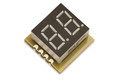•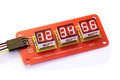•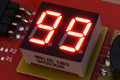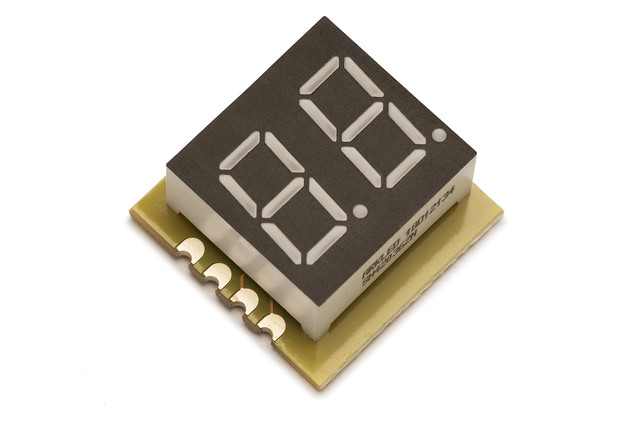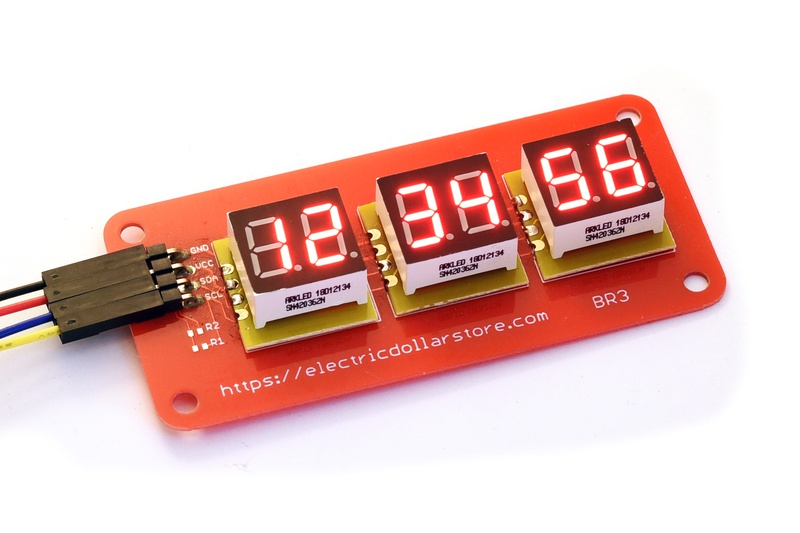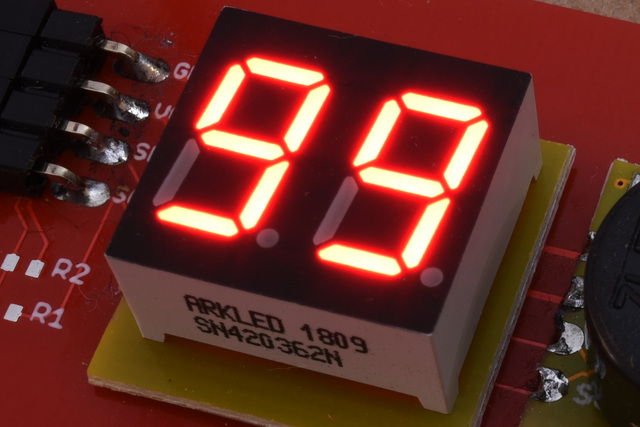• ## I²C 2 digit display

• bright red 8-segment display
• decimal/hex number display, and direct segment control
• dimmable in 255 gamma-corrected steps
• standard 18x18mm module with I²C and power

• \$1.50

DIG2 can be used it for indicators and readouts. It can be set to one of four I²C adresses, so multiple units can be used to make a display of up to 8 digits. The display is bright and flicker free. The brightness is software-controlled, and is gamma-corrected for a smooth increase over the entire 1-255 value range. DIG2 has a straightforward write-only protocol, with several commands for setting the display. The raw command sets all 16 segments:

0x00
<dig0>
<dig1>

The hex command displays a hex byte value 0x00-0xff:

0x01
<value>

The dec command displays a hex byte value 0-99:

0x02
<value>

The brightness command sets the brightness from 0x00 (completely dark) to 0xff (full brightness):

0x04
<bright>

```import sys
import serial
import time

from i2cdriver import I2CDriver, EDS

if __name__ == '__main__':
i2 = I2CDriver(sys.argv)

d = EDS.Dig2(i2)
for i in range(100):
d.dec(i)
time.sleep(.05)
```
```#include <Wire.h>

void dig2_show(byte v, int m = DEC)
{
Wire.beginTransmission(0x14);
Wire.write((m == HEX) ? 1 : 2);
Wire.write(v);
Wire.endTransmission();
}

void setup() {
Wire.begin();
}

int i;
void loop() {
dig2_show(i++);
delay(100);
}
```
```from machine import I2C
import time

class Dig2:
""" DIG2 is a 2-digit 7-segment LED display """

def __init__(self, i2, a = 0x14):
self.i2 = i2
self.a = a

def raw(self, b0, b1):
""" Set all 8 segments from the bytes b0 and b1 """
self.i2.writeto(self.a, bytes((0, b0, b1)))

def hex(self, b):
""" Display a hex number 0-0xff """
self.i2.writeto(self.a, bytes((1, b)))

def dec(self, b):
""" Display a decimal number 00-99 """
self.i2.writeto(self.a, bytes((2, b)))

def dp(self, p0, p1):
""" Set the state the decimal point indicators """
self.i2.writeto(self.a, bytes((3, (p1 << 1) | p0)))

def brightness(self, b):
""" Set the brightness from 0 (off) to 255 (maximum) """
self.i2.writeto(self.a, bytes((4, b)))

def main():
i2 = I2C(1, freq = 100000)

d = Dig2(i2)
for i in range(100):
d.dec(i)
time.sleep(.05)
```

 Default I²C address `0x14` (`0b0010100`) Current consumption (typ.) 25 mA Vcc 3.3 V